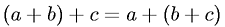Equations > Algebra > Addition/Subtraction > Associative Property of Addition

### Associative Property of AdditionLatex Code:

MathML Code:

 $\left(a+b\right)+c=a+\left(b+c\right)$

MathType 5.0: